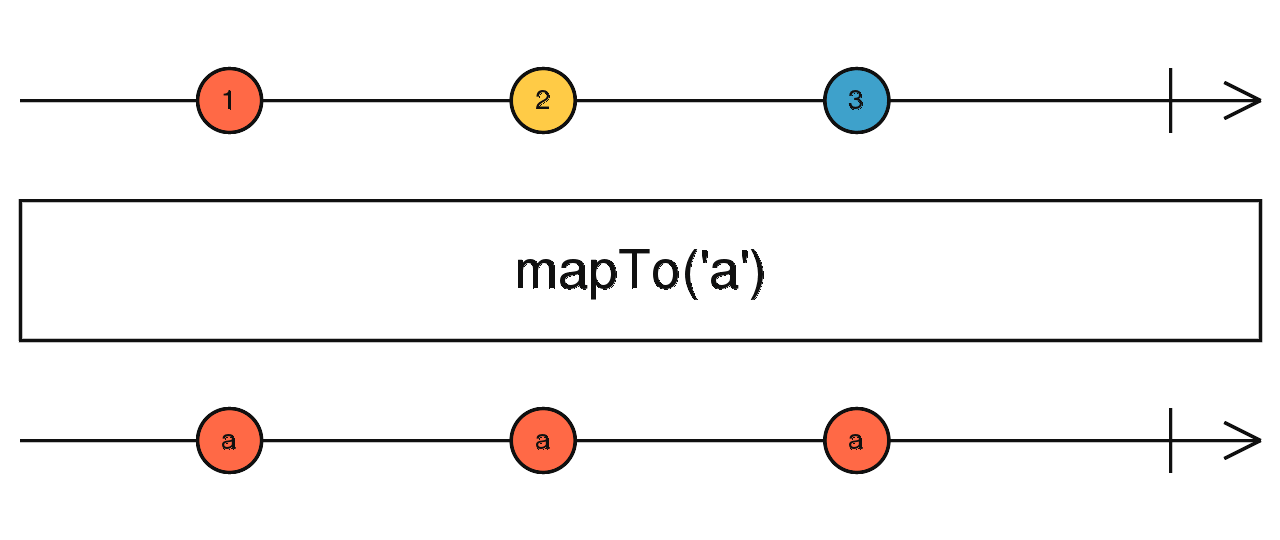/RxJS

# mapTo

`function` `stable`

Emits the given constant value on the output Observable every time the source Observable emits a value.

### `mapTo<T, R>(value: R): OperatorFunction<T, R>`

#### Parameters

 value The value to map each source value to.

#### Returns

`OperatorFunction<T, R>`: An Observable that emits the given `value` every time the source Observable emits something.

## Description

Like `map`, but it maps every source value to the same output value every time.Takes a constant `value` as argument, and emits that whenever the source Observable emits a value. In other words, ignores the actual source value, and simply uses the emission moment to know when to emit the given `value`.

## Example

Map every click to the string 'Hi'

```import { fromEvent } from 'rxjs';
import { mapTo } from 'rxjs/operators';

const clicks = fromEvent(document, 'click');
const greetings = clicks.pipe(mapTo('Hi'));
greetings.subscribe(x => console.log(x));```